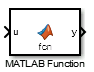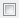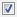# MATLAB Function

Include MATLAB code in models that generate embeddable C code

•Libraries:
HDL Coder / User-Defined Functions

## Description

With a MATLAB Function block, you can write a MATLAB® function for use in a Simulink® model. The MATLAB function executes for simulation and generates code for a Simulink Coder™ target. If you are new to Simulink and MATLAB products, see Implement MATLAB Functions in Simulink with MATLAB Function Blocks for an overview.

Double-clicking the MATLAB Function block opens the MATLAB Function Block Editor, where you write the MATLAB function. The example model `call_stats_block2` discussed in Implement MATLAB Functions in Simulink with MATLAB Function Blocks uses the following function in the MATLAB Function Block Editor:

```function [mean,stdev] = stats(vals) % Calculates a statistical mean and a standard % deviation for the values in vals. len = length(vals); mean = avg(vals,len); stdev = sqrt(sum(((vals-avg(vals,len)).^2))/len); plot(vals,"-+"); function mean = avg(array,size) mean = sum(array)/size;```

The function specifies the input and output data in the function declaration statement as arguments and return values. The argument and return values of the preceding example function correspond to the inputs and outputs of the block in the `call_stats_block2` model.You can also define variables, add an input trigger, and create function call outputs by using the Model Explorer or the Symbols pane. For more information, see Create and Define MATLAB Function Block Variables, Manage the Input Trigger of a MATLAB Function Block, and Manage Function Call Outputs of a MATLAB Function Block.

The MATLAB Function block generates efficient embeddable code based on an analysis that determines the size, class, and complexity of each variable. This analysis imposes the following restrictions:

• The first assignment to a variable defines its, size, class, and complexity.

• You cannot reassign variable properties after the initial assignment except when using variable-size data or reusing variables in the code for different purposes.

In addition to language restrictions, the MATLAB Function block supports a subset of the functions available in MATLAB. These functions include functions in common categories, such as:

For more information, see Functions and Objects Supported for C/C++ Code Generation.

Note

Although the code for this block attempts to produce exactly the same results as MATLAB, differences might occur due to rounding errors. These numerical differences, which might be a few `eps` initially, can magnify after repeated operations. Reliance on the behavior of `nan` is not recommended. Different C compilers can yield different results for the same computation.

Note

New MATLAB Function blocks do not include the `%#codegen` directive, but check for errors as if it is included. Adding the `%#codegen` directive to a MATLAB Function block does not affect error checking. For more information see Compilation Directive %#codegen.

To support visualization of data, the MATLAB Function block supports calls to MATLAB functions for simulation only. See Use MATLAB Engine to Execute a Function Call in Generated Code to understand some of the limitations of this capability, and how it integrates with code analysis for this block. If these function calls do not directly affect any of the Simulink inputs or outputs, the calls do not appear in Simulink Coder generated code.

From MATLAB Function blocks, you can also call functions defined in a Simulink Function block. You can call Stateflow® functions with Export Chart Level Functions (Make Global) and Allow exported functions to be called by Simulink checked in the chart Properties dialog box.

In the Symbols pane, you can declare a block input to be a Simulink parameter instead of a port. The MATLAB Function block also supports inheritance of types and size for inputs, outputs, and parameters. You can also specify these properties explicitly. See Define and Modify Variable Data Types, Specify Size of MATLAB Function Block Variables, and Configure MATLAB Function Block Parameter Variables for descriptions of variables that you use in MATLAB Function blocks.

Recursive calls are not allowed in MATLAB Function blocks.

By default, MATLAB Function blocks have direct feedthrough enabled. To disable it, clear the Allow direct feedthrough property. Nondirect feedthrough semantics ensure that outputs rely only on the current state. Using nondirect feedthrough enables you to use MATLAB Function blocks in a feedback loop and prevent algebraic loops. For more information, see Use Nondirect Feedthrough in a MATLAB Function Block.

## Ports

### Input

expand all

Input port, specified as a scalar, vector, or matrix. Each input variable that you create has a corresponding input port.

Data types supported by MATLAB but not supported by Simulink may not be passed between the Simulink model and the function within the MATLAB Function block. These types may be used within the MATLAB Function block.

For more information on fixed-point support for this block, refer to Fixed-Point Data Types with MATLAB Function Block (Fixed-Point Designer) and Data Type Override with MATLAB Function Block (Fixed-Point Designer).

#### Dependencies

To create input ports, open the block and create input variables in the Symbols pane. See Create and Define MATLAB Function Block Variables.

Data Types: `single` | `double` | `int8` | `int16` | `int32` | `int64` | `uint8` | `uint16` | `uint32` | `uint64` | `Boolean` | `string` | `fixed point` | `enumerated` | `bus`

### Output

expand all

Output port, specified as a scalar, vector, or matrix. Each output variable that you create has a corresponding output port.

#### Dependencies

To create output ports, open the block and create output variables in the Symbols pane. See Create and Define MATLAB Function Block Variables.

Data Types: `single` | `double` | `int8` | `int16` | `int32` | `int64` | `uint8` | `uint16` | `uint32` | `uint64` | `Boolean` | `string` | `fixed point` | `enumerated` | `bus`

## Parameters

expand all

### Main

Select how to display port labels on the MATLAB Function block icon.

• `none` – Do not display port labels.

• `FromPortIcon` – Display the name of the input and output variables.

• `FromPortBlockName` – Display the name of the input and output variables.

• `SignalName` – If the signal connected to the port is named, display the signal name. Otherwise, display the name of the variables.

#### Programmatic Use

 Parameter: `ShowPortLabels` Type: string scalar or character vector Value: `"none"` | `"FromPortIcon"` | `"FromPortBlockName"` | `"SignalName"` Default: `"FromPortIcon"`

• `ReadWrite` – Enable opening and modifying of MATLAB Function block contents.

• `ReadOnly` – Enable opening of the MATLAB Function block.

• `NoReadOrWrite` – Disable opening or modifying of the MATLAB Function block.

Note

When you attempt to view the contents of a MATLAB Function block whose Read/Write permissions parameter is `NoReadOrWrite`, the block does not respond. For example, when you double-click the MATLAB Function block, Simulink does not open the table contents and does not display messages.

#### Programmatic Use

 Parameter: `Permissions` Type: string scalar or character vector Value: `"ReadWrite"` | `"ReadOnly"` | `"NoReadOrWrite"` Default: `"ReadWrite"`

Whether the block attempts to eliminate artificial algebraic loops that include the atomic unit during simulation.

•off – Do not try to eliminate any artificial algebraic loops that include the atomic unit.

•on – Try to eliminate any artificial algebraic loops that include the atomic unit.

#### Programmatic Use

 Parameter: `MinAlgLoopOccurrences` Type: string scalar or character vector Value: `"off"` | `"on"` Default: `"off"`

Specify whether variables in this block must run at the same rate or can run at different rates.

• If variables in the MATLAB Function block can run at different rates, specify the sample time as inherited (`-1`).

• If variables must run at the same rate, specify the sample time, `Ts`, corresponding to this rate.

#### Programmatic Use

 Parameter: `SystemSampleTime` Type: string scalar or character vector Value: `"-1"` | `"[Ts 0]"` Default: `"-1"`

### Code Generation

Select the code format that the block generates.

`Auto`

Simulink Coder chooses the optimal format for your system based on the type and number of instances of the MATLAB Function block that exist in the model.

`Inline`

Simulink Coder inlines the MATLAB Function block unconditionally.

`Nonreusable function`

Simulink Coder explicitly generates a separate function in a separate file. MATLAB Function blocks with this setting generate functions that might have arguments depending on the Function interface in Code Generation. parameter setting. You can name the generated function and file using parameters Function name and File name (no extension), respectively from Code Generation. These functions are not reentrant.

`Reusable function`

Simulink Coder generates a function with arguments that allows reuse of MATLAB Function block code when a model includes multiple instances of the MATLAB Function block.

This option generates a function with arguments that allows MATLAB Function block code to be reused in the generated code of a model reference hierarchy that includes multiple instances of a MATLAB Function block across referenced models. In this case, the MATLAB Function block must be in a library.

#### Tips

• When you want to represent multiple instances of a MATLAB Function block as one reusable function, you can designate each of the instances as `Auto` or as `Reusable function`. It is best to use one or the other, as using both creates two reusable functions, one for each designation. The outcomes of these choices differ only when reuse is not possible. Selecting `Auto` does not allow control of the function or file name for the MATLAB Function block code.

• The `Reusable function` and `Auto` options both determine whether multiple instances of a MATLAB Function block exist and the code can be reused. The options behave differently when it is impossible to reuse the code. In this case, `Auto` yields inlined code, or if circumstances prohibit inlining, separate functions for each block instance.

• If you select `Reusable function` while your generated code is under source control, set File name options to `Use subsystem name`, `Use function name`, or `User specified`. Otherwise, the names of your code files change when you modify your model, which prevents source control on your files.

#### Dependency

• This parameter requires Simulink Coder.

• Setting this parameter to ```Nonreusable function``` or ```Reusable function``` enables the following parameters:

• Function name options

• File name options

• Memory section for initialize/terminate functions (requires Embedded Coder® and an ERT-based system target file)

• Memory section for execution functions (requires Embedded Coder and an ERT-based system target file)

• Setting this parameter to ```Nonreusable function``` enables Function with separate data (requires a license for Embedded Coder and an ERT-based system target file).

#### Programmatic Use

 Parameter: `RTWSystemCode` Type: string scalar or character vector Value: `"Auto"` | `"Inline"` | `"Nonreusable function"` | `"Reusable function"` Default: `"Auto"`

## Block Characteristics

 Data Types `Booleana` | `busa` | `doublea` | `enumerateda` | `fixed pointa` | `halfa` | `integera` | `singlea` | `stringa` Direct Feedthrough `yesa` Multidimensional Signals `yesa` Variable-Size Signals `yesa` Zero-Crossing Detection `no` a Actual data type or capability support depends on block implementation.

## Version History

Introduced in R2011a

expand all